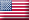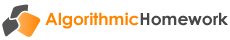# Financial Mathematics Edition 2Introduces the mathematical tools that are used in finance and develops a command of business calculations by providing unlimited practice of real world applications. The algebraic formulas of discrete financial mathematics are revealed, explained and used directly to perform calculations. The purpose of this e-workbook is to facilitate a strong understanding of financial mathematics and its application to a wide range of transactions.

#### Fundamental concepts

• Concepts of risk and return
• The time value of money
• Positioning of cash flows on a timeline

#### Simple interest and simple discount

• Calculating the amount of simple interest
• Calculating the number of days between dates
• Future value at simple interest
• Present value at simple interest
• Finding the interest rate and time period
• Promissory notes at simple interest
• Promissory notes at simple discount

#### Compound interest

• Nominal, effective and equivalent rates
• Future value at compound interest
• Present value at compound interest
• Finding the interest rate and time period
• Equations of value
• Changing interest rates

#### Annuities

• Future value of an ordinary annuity
• Present value of an ordinary annuity
• Present value of a deferred annuity
• Present value of a perpetuity
• Finding the periodic payment

#### More annuity problems

• Annuities due: valuation and periodic payment
• Finding the interest rate of an annuity
• Finding the term of an annuity
• Valuation under conditions of changing rates

#### Applications to personal finance

• Finding the periodic payment for a housing loan
• Calculating a loan repayment schedule
• Retrospective and prospective methods of loan outstanding
• Adjusting the repayment amount in response to changes in the loan interest rate
• Adjusting the term in response to changes in the loan interest rate
• Applications of business mathematics to bank accounts and credit cards

#### Applications to corporate finance

• Pricing a bond on a coupon date
• Pricing a bond between coupon dates
• Solving for the yield to maturity implied in a bond purchase
• Calculating the holding period return from a bond trade
• Allowing for income and capital gains tax on bonds
• Simple share valuation
• Share valuation allowing for dividend growth
• Making investment decisions based upon net present value
• Assessing corporate projects using the internal rate of return
• Calculating costs of funds for corporate debt
• Raising floating rate funds through bill facilities